《天龍八部》一直是我最喜歡的金庸著作之一，最近重新翻閱，有很多新的感受。## 生成新的天龍八部橋段¶

烏老大偏生要考一考慕容復，說道：「慕容公子，你瞧這不是大大的


不算？」馬夫人道：「不錯，咱們非要尋死不可。」（建議在網速快的地方載入模型以減少等待時間，或者點擊載入後先閱讀模型是怎麼被訓練的，等等再回來查看）

• 生成文章來讓模型依據同輸入產生新橋段
• 重置輸入來隨機取得一個新的起始句子
• 增加模型生成的文章長度
• 調整生成溫度來改變文章的變化性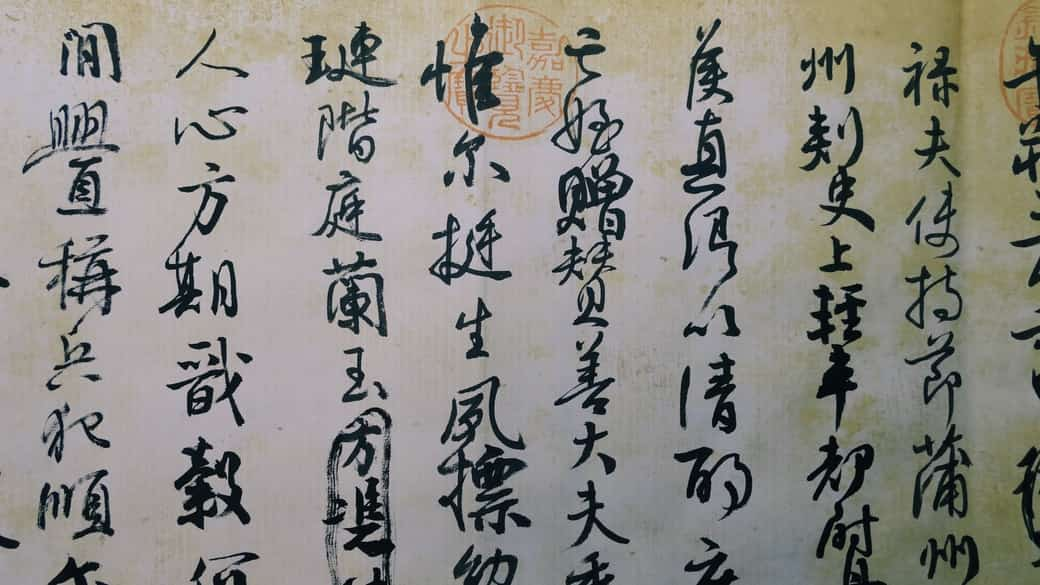## 模型是怎麼被訓練的¶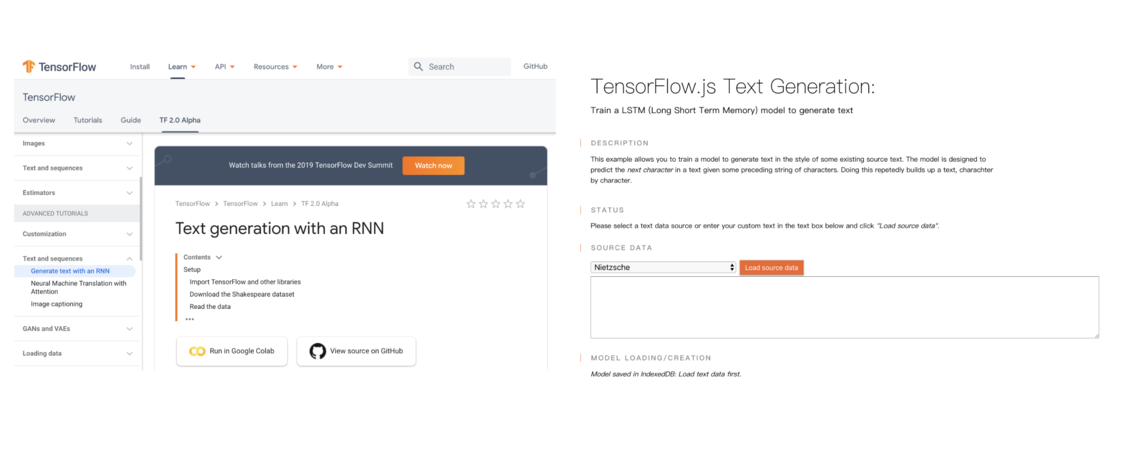## TensorFlow 2.0 開發¶

TensorFlow 2.0 值得關注的更新不少，但以下幾點跟一般的 ML 開發者最為相關：

pip install tf-nightly-gpu-2.0-preview


## 深度學習專案步驟¶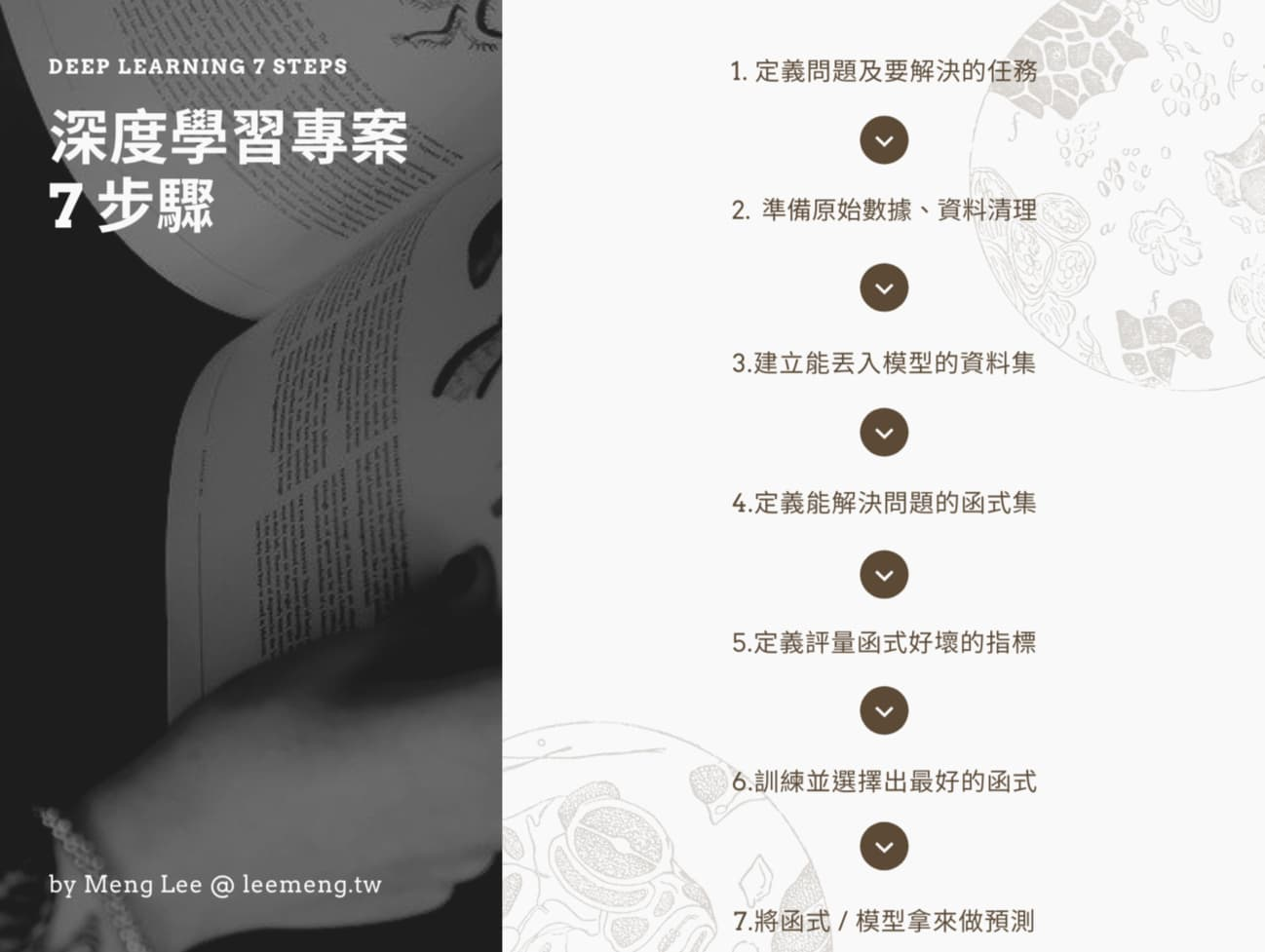### 1. 定義問題及要解決的任務¶

• 字（Character，如劍、寺、雲）
• 詞（Word，如吐蕃、師弟、阿修羅）

『六脈神劍經』乃本寺鎮寺之寶，大理段氏武學的至高法要。


『六脈神劍經』乃本寺鎮寺之寶，大理段氏武


### 2. 準備原始數據、資料清理¶# 隨意取出第 9505 到 9702 的中文字
print(text[9505:9702])

咱們見敵方人多，不得師父號令，沒敢隨便動手。」左子穆道：「嗯，來了多少人？」干光豪道：「大約七八十人。」左子穆嘿嘿冷笑，道：「七八十人，便想誅滅無量劍了？只怕也沒這麼容易。」



n = len(text)
w = len(set(text))
print(f"天龍八部小說共有 {n} 中文字")
print(f"包含了 {w} 個獨一無二的字")

天龍八部小說共有 1235431 中文字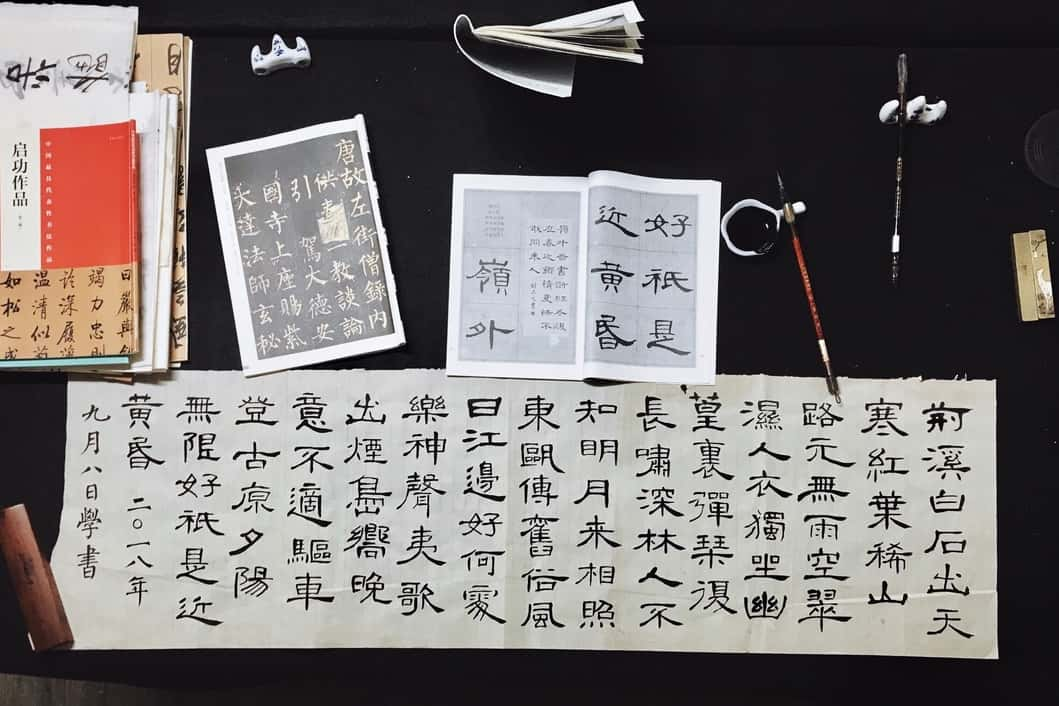import tensorflow as tf

# 初始化一個以字為單位的 Tokenizer
tokenizer = tf.keras\
.preprocessing\
.text\
.Tokenizer(
num_words=num_words,
char_level=True,
filters=''
)

# 讓 tokenizer 讀過天龍八部全文，
# 將每個新出現的字加入字典並將中文字轉
# 成對應的數字索引
tokenizer.fit_on_texts(text)
text_as_int = tokenizer\
.texts_to_sequences([text])

# 隨機選取一個片段文本方便之後做說明
s_idx = 21004
e_idx = 21020
partial_indices = \
text_as_int[s_idx:e_idx]
partial_texts = [
tokenizer.index_word[idx] \
for idx in partial_indices
]

# 渲染結果，可忽略
print("原本的中文字序列：")
print()
print(partial_texts)
print()
print("-" * 20)
print()
print("轉換後的索引序列：")
print()
print(partial_indices)

原本的中文字序列：

['司', '空', '玄', '雙', '掌', '飛', '舞', '，', '逼', '得', '牠', '無', '法', '近', '前', '。']

--------------------

[557, 371, 215, 214, 135, 418, 1209, 1, 837, 25, 1751, 49, 147, 537, 111, 2]


人類看的中文字   機器看的輸入索引
------------------------------

，                  1

。                  2


### 3. 建立能丟入模型的資料集¶

text_as_int[:10]

[1639, 148, 3, 3, 280, 5, 192, 819, 374, 800]
_type = type(text_as_int)
n = len(text_as_int)
print(f"text_as_int 是一個 {_type}\n")
print(f"小說的序列長度： {n}\n")
print("前 5 索引：", text_as_int[:5])

text_as_int 是一個 <class 'list'>



print("實際丟給模型的數字序列：")
print(partial_indices[:-1])
print()
print("方便我們理解的文本序列：")
print(partial_texts[:-1])

實際丟給模型的數字序列：
[557, 371, 215, 214, 135, 418, 1209, 1, 837, 25, 1751, 49, 147, 537, 111]

['司', '空', '玄', '雙', '掌', '飛', '舞', '，', '逼', '得', '牠', '無', '法', '近', '前']


print("實際丟給模型的數字序列：")
print(partial_indices[1:])
print()
print("方便我們理解的文本序列：")
print(partial_texts[1:])

實際丟給模型的數字序列：
[371, 215, 214, 135, 418, 1209, 1, 837, 25, 1751, 49, 147, 537, 111, 2]

['空', '玄', '雙', '掌', '飛', '舞', '，', '逼', '得', '牠', '無', '法', '近', '前', '。']司 空 玄 雙 掌 飛 舞 ， 逼 得 牠 無 法 近



• 看到第一個輸入字 司 時可以正確輸出 空
• 在之前看過 司，且新輸入字為 空 的情況下，可以輸出 玄
• 在之前看過 司空，且新輸入字為 玄 的情況下，可以輸出 雙
• 在之前看過 司空玄雙掌飛，且新輸入字為 舞 的情況下，可以輸出 ，時間點 輸入字  輸入索引   輸出字  輸出索引
-------------------------------------
1    司     557      空      371
2    空     371      玄      215
3    玄     215      雙      214
4    雙     214      掌      135
5    掌     135      飛      418
6    飛     418      舞      1209
7    舞     1209     ，      1
8    ，     1        逼      837
9    逼     837      得      25
10    得     25       牠      1751
11    牠     1751     無      49
12    無     49       法      147
13    法     147      近      537
14    近     537      前      111


• 輸入索引代表模型在當下時間吃進去的輸入
• 輸出索引則代表我們要模型輸出的結果# 方便說明，實際上我們會用更大的值來
# 讓模型從更長的序列預測下個中文字
SEQ_LENGTH = 10  # 數字序列長度
BATCH_SIZE = 128 # 幾筆成對輸入/輸出

# text_as_int 是一個 python list
# 我們利用 from_tensor_slices 將其
# 轉變成 TensorFlow 最愛的 Tensor <3
characters = tf\
.data\
.Dataset\
.from_tensor_slices(
text_as_int)

# 將被以數字序列表示的天龍八部文本
# 拆成多個長度為 SEQ_LENGTH (10) 的序列
# 並將最後長度不滿 SEQ_LENGTH 的序列捨去
sequences = characters\
.batch(SEQ_LENGTH + 1,
drop_remainder=True)

# 天龍八部全文所包含的成對輸入/輸出的數量
steps_per_epoch = \
len(text_as_int) // SEQ_LENGTH

# 這個函式專門負責把一個序列
# 拆成兩個序列，分別代表輸入與輸出
# （下段有 vis 解釋這在做什麼）
def build_seq_pairs(chunk):
input_text = chunk[:-1]
target_text = chunk[1:]
return input_text, target_text

# 將每個從文本擷取出來的序列套用上面
# 定義的函式，拆成兩個數字序列
# 作為輸入／輸出序列
# 再將得到的所有數據隨機打亂順序
# 最後再一次拿出 BATCH_SIZE（128）筆數據
# 作為模型一次訓練步驟的所使用的資料
ds = sequences\
.map(build_seq_pairs)\
.shuffle(steps_per_epoch)\
.batch(BATCH_SIZE,
drop_remainder=True)擷取的片段序列       輸入/輸出序列
-------------------------------
-> 烏老大拱手還
|

|
-> 老大拱手還禮

-> 星宿派人數遠
|

|
-> 宿派人數遠較

-> 過不多時，賈
|

|
-> 不多時，賈老TensorFlow 2.0 預設就是 Eager Execution，因此你不再需要使用老朋友 tf.Session() 或是 tf.placeholder 就能非常直覺地存取數據：

# print 是用來幫你理解 tf.data.Dataset
# 的內容，實際上存取資料集非常簡單
# 現在先關注下面的 print 結果
for b_inp, b_tar in ds.take(1):
print("起始句子的 batch：")
print(b_inp, "\n")
print("目標句子的 batch：")
print(b_tar, "\n")
print("-" * 20, "\n")

print("第一個起始句子的索引序列：")
first_i = b_inp.numpy()
print(first_i, "\n")
print("第一個目標句子的索引序列：")
first_t = b_tar.numpy()
print(first_t, "\n")
print("-" * 20, "\n")

d = tokenizer.index_word
print("第一個起始句子的文本序列：")
print([d[i] for i in first_i])
print()
print("第一個目標句子的文本序列：")
print([d[i] for i in first_t])

起始句子的 batch：
tf.Tensor(
[[1440   10   12 ... 1882   15  175]
[ 157   16  212 ...   11  206   92]
[  36   14   36 ...  368  384   63]
...
[  61    8    3 ...   11    5  219]
[ 123  189  587 ...   65  120   51]
[   1    5  620 ...    2    8 1272]], shape=(128, 10), dtype=int32)

tf.Tensor(
[[  10   12    7 ...   15  175   99]
[  16  212   67 ...  206   92    1]
[  14   36   36 ...  384   63    2]
...
[   8    3    3 ...    5  219    1]
[ 189  587  884 ...  120   51  196]
[   5  620  597 ...    8 1272 1275]], shape=(128, 10), dtype=int32)

--------------------

[1440   10   12    7   63   19   17 1882   15  175]

[  10   12    7   63   19   17 1882   15  175   99]

--------------------

['陵', '道', '：', '「', '想', '來', '他', '嫌', '你', '本']

['道', '：', '「', '想', '來', '他', '嫌', '你', '本', '事']


for b_inp, b_tar in ds.take(1):
# 蒙多想去哪就去哪
# 想怎麼存取 b_iup, b_tar 都可以
print("b_inp 是個 Tensor：\n")
print(b_inp)
print("\nb_tar 也是個 Tensor，")
print("只是每個數字序列都是"
"對應的輸入序列往左位"
"移一格的結果\n")
print(b_tar)

b_inp 是個 Tensor：

tf.Tensor(
[[   2  953 1214 ...    1   52  219]
[   6    2   15 ...   36  189    5]
[2456 1167 3142 ...  110 1186   56]
...
[ 422  244   19 ...    2    8   46]
[ 254   51  237 ...  123   64   27]
[1561   25   55 ...   66    2    3]], shape=(128, 10), dtype=int32)

b_tar 也是個 Tensor，

tf.Tensor(
[[ 953 1214   41 ...   52  219   52]
[   2   15  189 ...  189    5  189]
[1167 3142 1294 ... 1186   56    5]
...
[ 244   19  145 ...    8   46   41]
[  51  237  202 ...   64   27  569]
[  25   55    9 ...    2    3    3]], shape=(128, 10), dtype=int32)


### 4. 定義能解決問題的函式集¶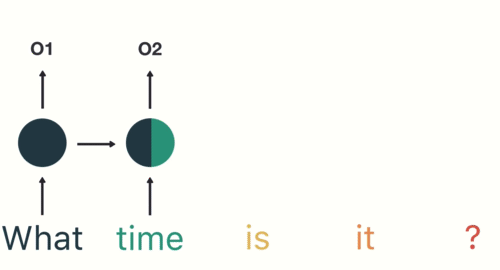# 超參數
EMBEDDING_DIM = 512
RNN_UNITS = 1024

# 使用 keras 建立一個非常簡單的 LSTM 模型
model = tf.keras.Sequential()

# 詞嵌入層
# 將每個索引數字對應到一個高維空間的向量
tf.keras.layers.Embedding(
input_dim=num_words,
output_dim=EMBEDDING_DIM,
batch_input_shape=[
BATCH_SIZE, None]
))

# LSTM 層
# 負責將序列數據依序讀入並做處理
tf.keras.layers.LSTM(
units=RNN_UNITS,
return_sequences=True,
stateful=True,
recurrent_initializer='glorot_uniform'
))

# 全連接層
# 負責 model 每個中文字出現的可能性
tf.keras.layers.Dense(
num_words))

model.summary()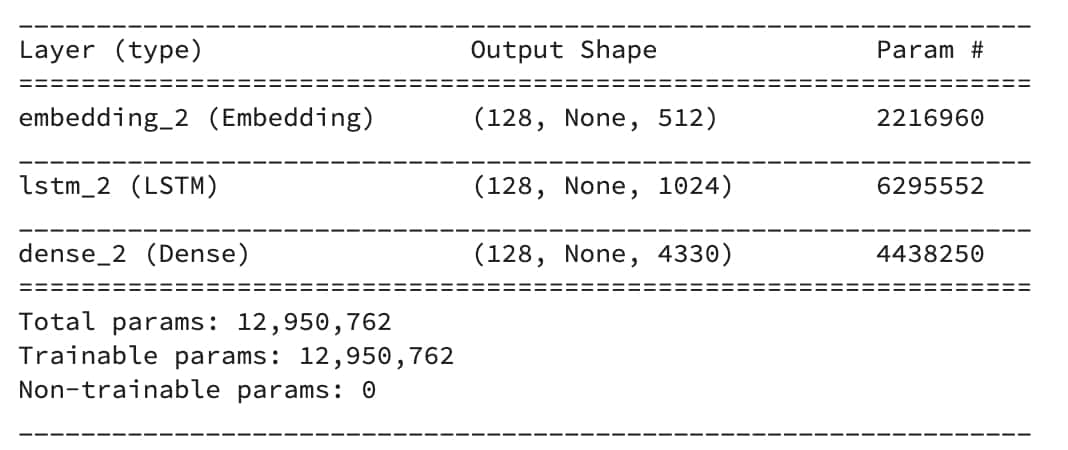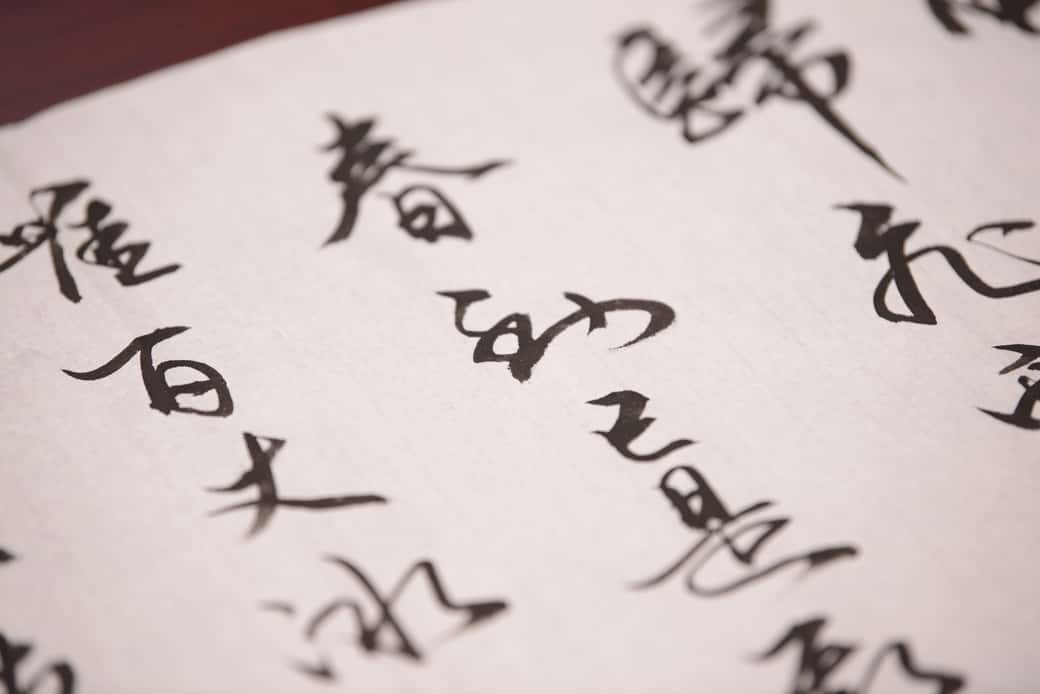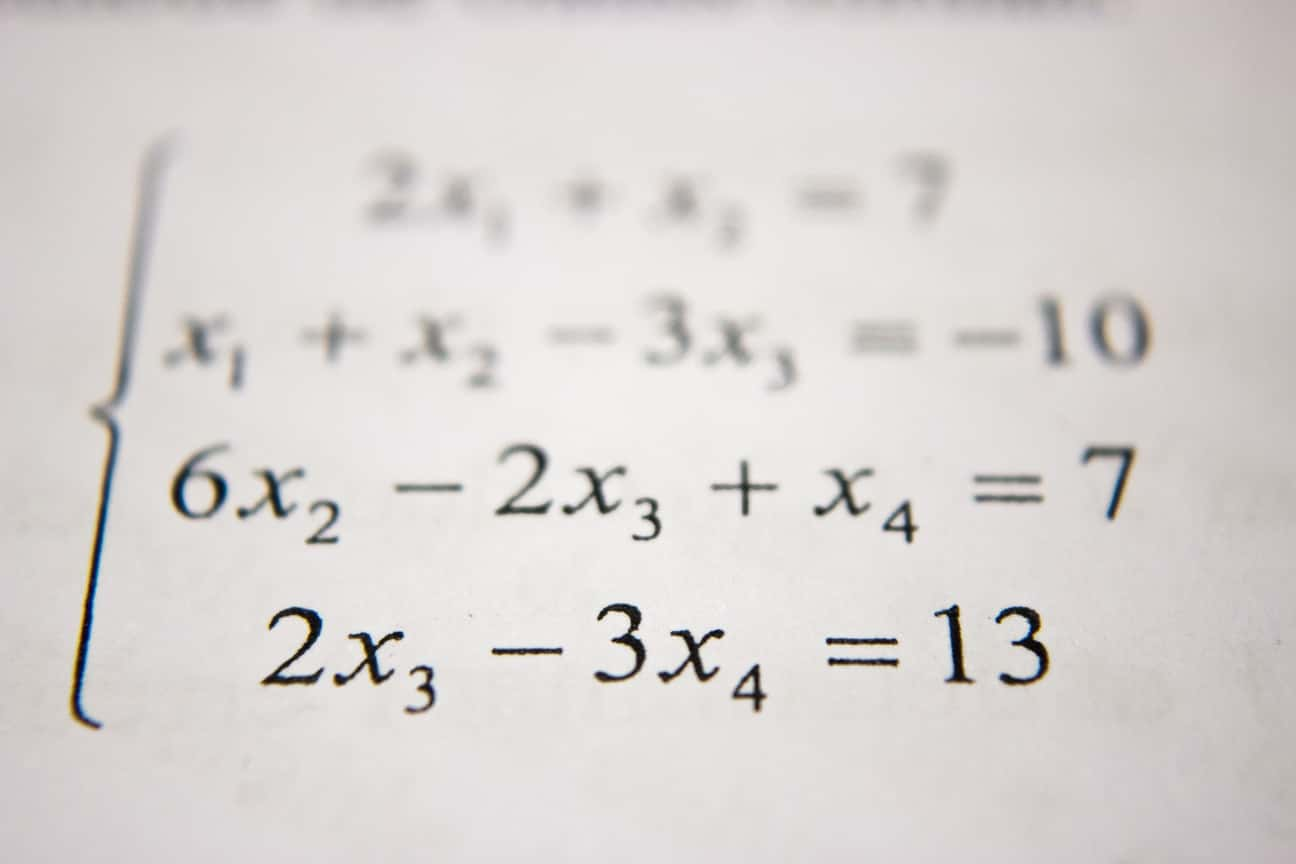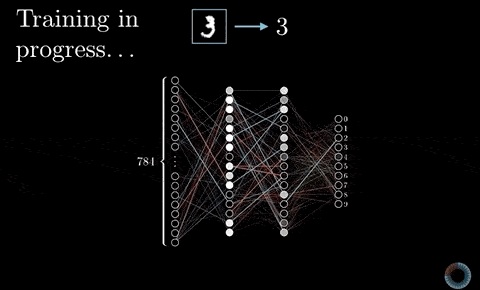### 5. 定義評量函式好壞的指標¶# 超參數，決定模型一次要更新的步伐有多大
LEARNING_RATE = 0.001

# 定義模型預測結果跟正確解答之間的差異
# 因為全連接層沒使用 activation func
# from_logits= True
def loss(y_true, y_pred):
return tf.keras.losses\
.sparse_categorical_crossentropy(
y_true, y_pred, from_logits=True)

# 剛剛定義的損失函數
model.compile(
optimizer=tf.keras\
learning_rate=LEARNING_RATE),
loss=loss
)


model.compile 讓我們告訴模型在訓練的時候該使用什麼優化器（optimizers）來最小化剛剛定義的損失函數

### 6. 訓練並選擇出最好的函式¶

EPOCHS = 10 # 決定看幾篇天龍八部文本
history = model.fit(
ds, # 前面使用 tf.data 建構的資料集
epochs=EPOCHS
)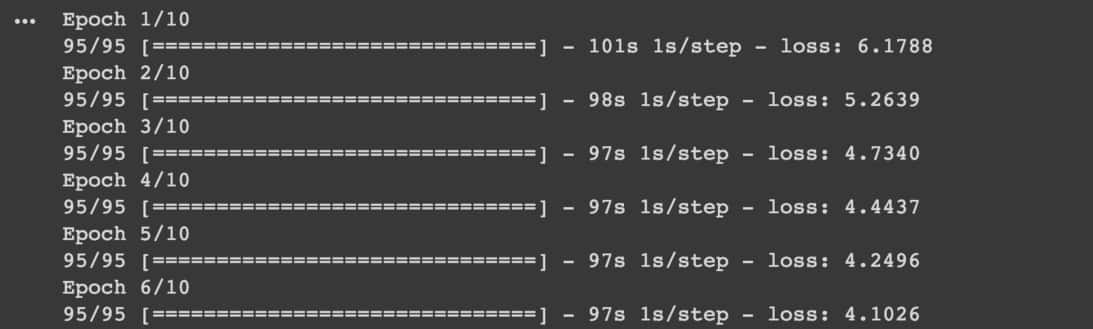Keras 模型在訓練時就會不斷吐出結果供你參考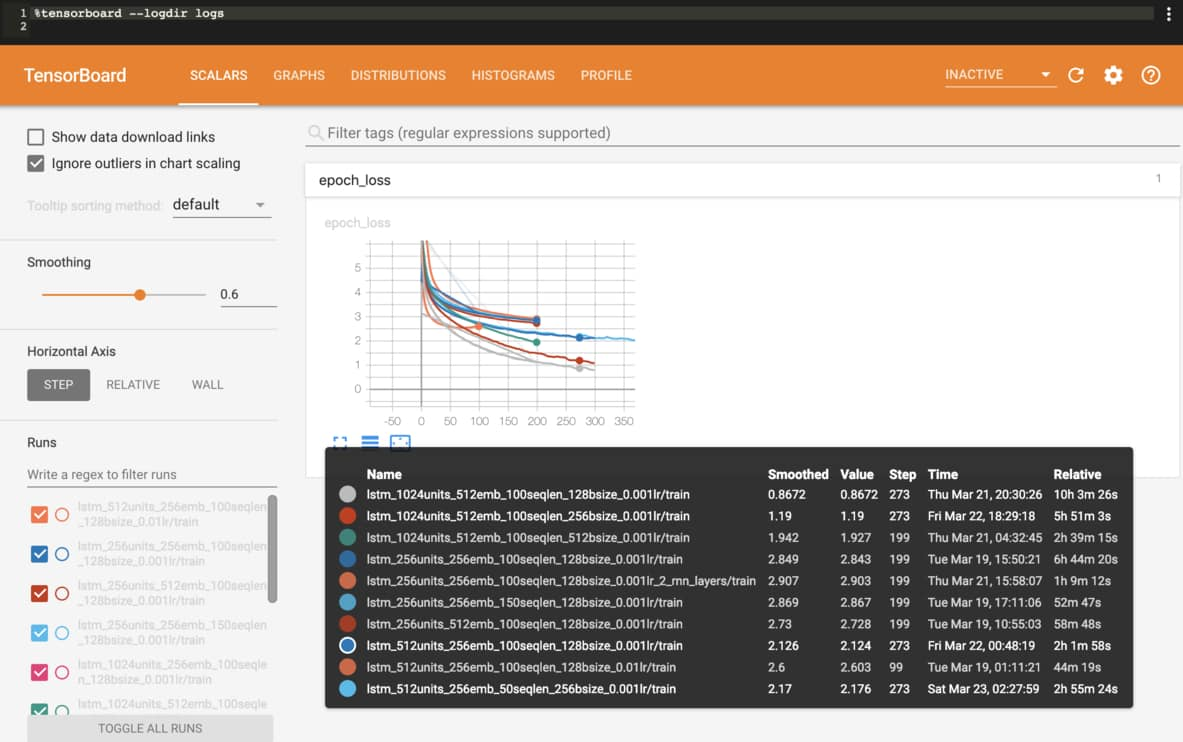TensorFlow 2.0 新增了 JupyterNotebook 的 Extension，讓你可以直接在筆記本或是 Google Colab 上邊訓練模型邊查看結果。

callbacks = [
tf.keras.callbacks\
.TensorBoard("logs"),
# 你可以加入其他 callbacks 如
# ModelCheckpoint,
# EarlyStopping
]

history = model.fit(
ds,
epochs=EPOCHS,
callbacks=callbacks
)


%load_ext tensorboard.notebook
%tensorboard --logdir logs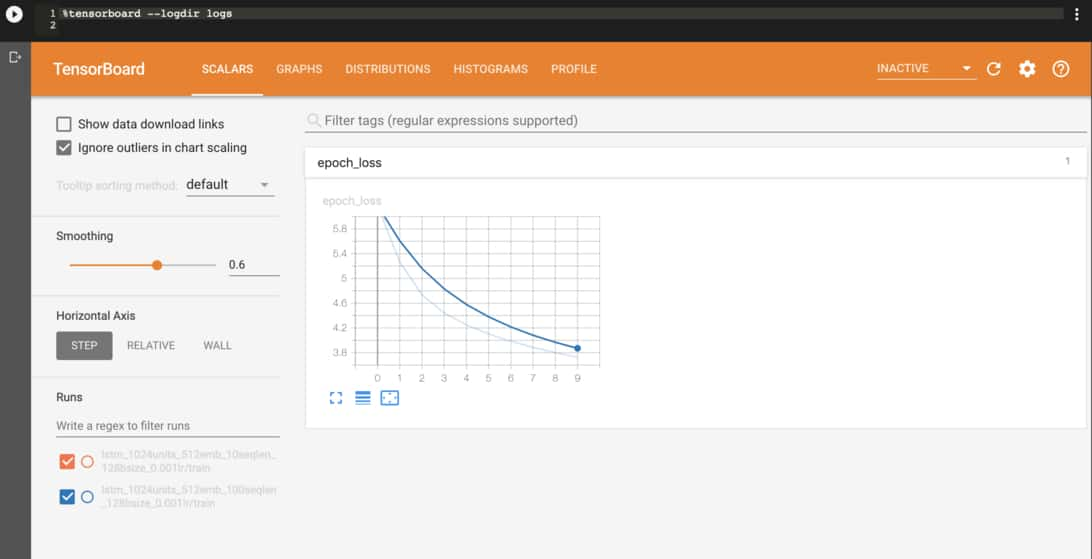喬峯指著深谷，


喬峯指著深谷，鑠淆孤癸抑私磚簧麥笠簸殯膽稼匿聲罪殖省膻臆啟殖
》斥酒燥弄咪薔鬃衝矚理蝗驗吞柢舌滴漂撿毛等櫈磁槃鞭爛辣諱輝母犢楊拜攜戛婉額虐延久鋒幟懸質迸飭南軌忸瑩娘檔麵獎逍菌包怖續敗倨凍赭彈暖顴衽劑街榻裝貨啕畿驛吳喬峯指著深谷，說道：「我不知道，不是你的好人，你就是你的好。」木婉清道：「他……你……我……我……師父是誰？」



• 模型已經知道怎麼產生正確的人名
• 知道 道 後面要接冒號以及上括號
• 知道有上括號時後面應該要有下括號
• 知道要適時加入換行

喬峯指著深谷，往前走去。



擤了些鼻涕拋下 很不段譽，但我還是笑了。### 7. 將函式 / 模型拿來做預測¶

# 跟訓練時一樣的超參數，
# 只差在 BATCH_SIZE 為 1
EMBEDDING_DIM = 512
RNN_UNITS = 1024
BATCH_SIZE = 1

# 專門用來做生成的模型
infer_model = tf.keras.Sequential()

# 詞嵌入層
tf.keras.layers.Embedding(
input_dim=num_words,
output_dim=EMBEDDING_DIM,
batch_input_shape=[
BATCH_SIZE, None]
))

# LSTM 層
tf.keras.layers.LSTM(
units=RNN_UNITS,
return_sequences=True,
stateful=True
))

# 全連接層
tf.keras.layers.Dense(
num_words))

# 讀入之前訓練時儲存下來的權重
infer_model.build(
tf.TensorShape([1, None]))


• 將起始文本丟入模型
• 抽樣得到新的中文字
• 將新得到的字再丟入模型
• 重複上述步驟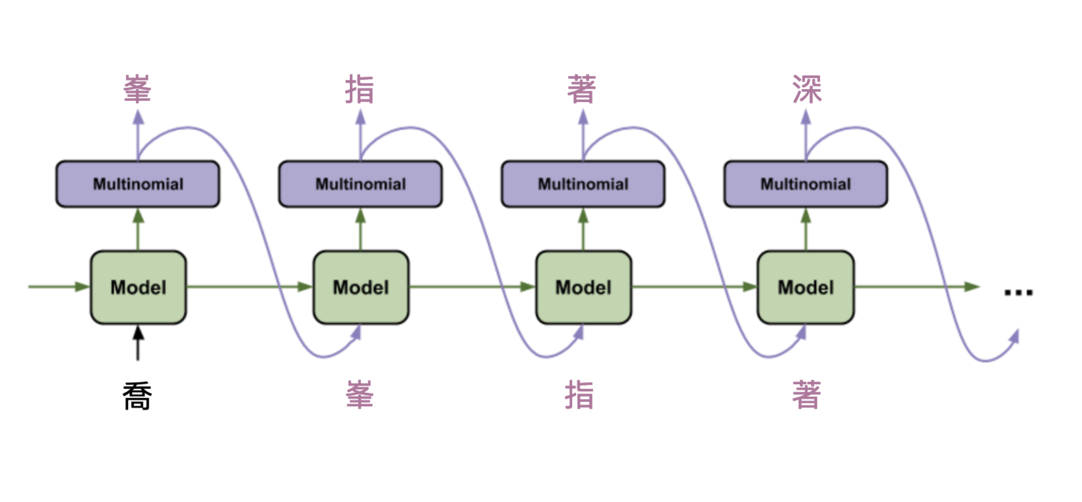# 代表「喬」的索引
seed_indices = 

# 增加 batch 維度丟入模型取得預測結果後
# 再度降維，拿掉 batch 維度
input = tf.expand_dims(
seed_indices, axis=0)
predictions = infer_model(input)
predictions = tf.squeeze(
predictions, 0)

# 利用生成溫度影響抽樣結果
predictions /= temperature

# 從 4330 個分類值中做抽樣
# 取得這個時間點模型生成的中文字
sampled_indices = tf.random\
.categorical(
predictions, num_samples=1)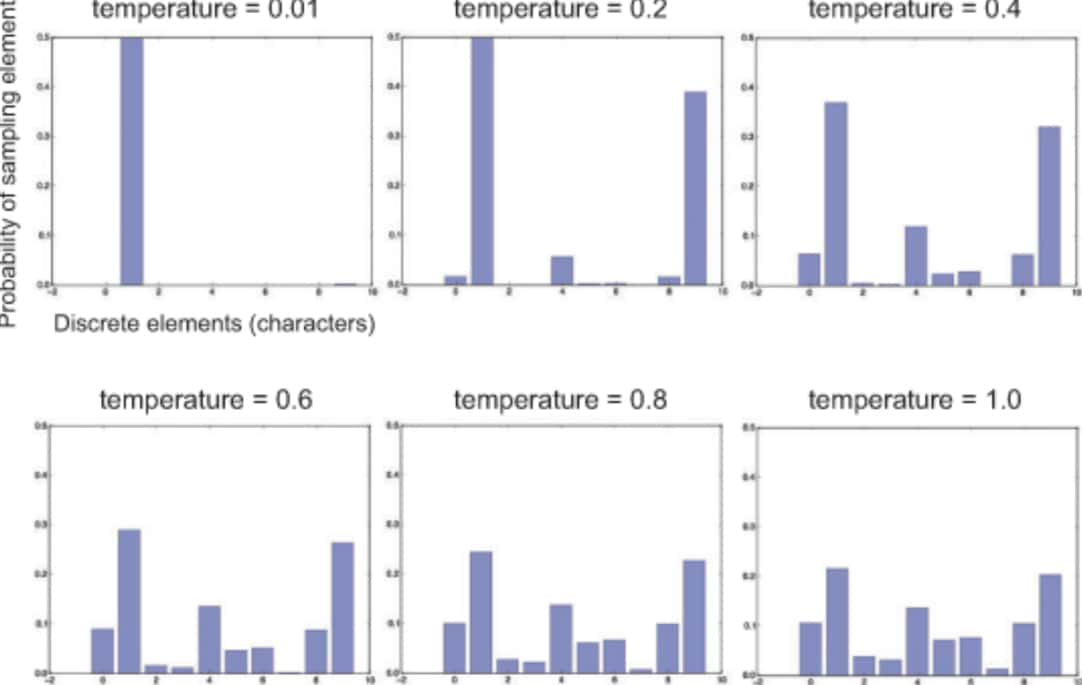## 如何使用 TensorFlow.js 跑模型並生成文章¶

infer_model.save("model.h5")


tensorflowjs_converter \
--input_format=keras \
model.h5 \
tfjs_model_folder


model = tf.loadLayersModel("url");
const output = model.predict(input);


• 隱私有保障。使用者上傳、輸入的內容不會被上傳到伺服器
• 開發者不需租借伺服器或是建置 API 端點，無部署成本

## 結語¶

• 如何利用深度學習 7 步驟開發 AI 應用
1. 定義問題及要解決的任務
2. 準備原始數據、資料清理
3. 建立能丟入模型的資料集
4. 定義能解決問題的函式集
5. 定義評量函式好壞的指標
6. 訓練並選擇出最好的函式
7. 將函式 / 模型拿來做預測
• 了解如何利用深度學習解決序列生成任務
• 熟悉 TensorFlow 2.0 的重要功能
• tf.keras
• tf.data
• TensorBoard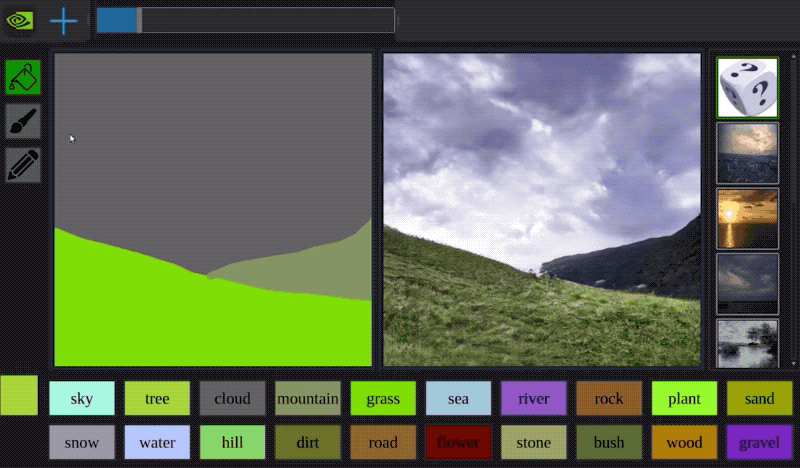GauGAN 能幫助一般人繪出美麗圖片，也能讓藝術家更快將點子實現出來 （圖片來源